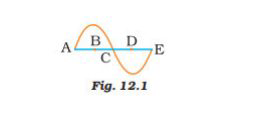# NCERT Exemplar Solutions Class 9 Science Solutions for Sound - Exercise in Chapter 12 - Sound

In the curve (Fig.12.1) half the wavelength is

(a) A B

(b) B D

(c) D E

(d) A EThe answer is (b) B D

Explanation:

Wavelength is the distance between two consecutive troughs. In the graph half, the wavelength will BD.

Video transcript
"hello everyone welcome to leader learning i am good preet your science tutor today's question is in the curve figure 12.1 here you can see a curve half the wavelength is option a a b option b b d option c d e or option d a e so here four options have been given to you and we have to find out that from the curve in the figure the half of the wavelength will be what so let's start our answer since wavelength is the distance between two consecutive troughs or crust okay and if you see in the figure in the graph the half of the wavelength will be bd okay so the correct option for this question is option b that is bd for more such videos please subscribe leader learning and for any doubts drop a comment thank you"
Related Questions
Exercises

Lido

Courses

Teachers

Book a Demo with us

Syllabus

Maths
CBSE
Maths
ICSE
Science
CBSE

Science
ICSE
English
CBSE
English
ICSE
Coding

Terms & Policies

Selina Question Bank

Maths
Physics
Biology

Allied Question Bank

Chemistry
Connect with us on social media!